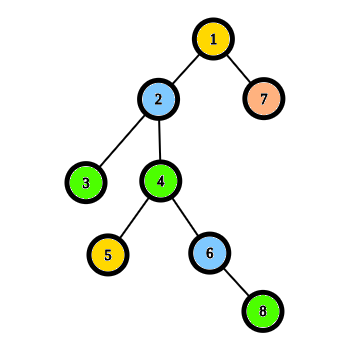# Chow, MorebornLong Practice Sessions on...
Limits 1s, 256 MB

Mo, the jumper has found an extraordinary tree in the woods. He absolutely loves it. He already has made quite a few jumps from one branch to another. After a while, being bored, he wonders what would be the minimum time to reach a vertex from another.

Formally, a tree is a connected graph with bidirectional edges and there exists only one path between two vertices. The tree Mo found is rooted at $1$ and has $N$ vertices numbered from $1$ to $N$. Every vertex $i$ has some color $c_i$. It takes him $d$ seconds to go from vertex $u$ to $v$ if $u, ~v$ are adjacent. Also, Mo can jump from a vertex $u$ to vertex $v$ if the colors of those two vertices are same, i.e. $c_u = c_v$. These jumps take him $f_{c_u}$ seconds.

For two vertices $s$ and $t$, find out the minimum time needed for Mo to reach $t$ from $s$.

## Input

First line contains an integer $T$.

There are $T$ test cases. For each test case ---

• First line contains two integers $N, ~d$, giving the number of vertices in the tree and the time needed to move between adjacent vertices. The vertices are numbered from $1$ to $N$.
• Next line follows with $N-1$ integers, the $i$th of which denotes the parent of vertex $i+1$.
• The next line contains $N$ integers, the $i$th integer denotes the color of vertex $i$, $c_i$.
• The following line contains another $N$ integers, the $i$th integer denotes $f_{c_i}$. It is guaranteed that for any $i, ~j$, if $c_i = c_j$ then $f_{c_i} = f_{c_j}$.
• The last line contains two integers $s$ and $t$, the source and the destination.

#### Scoring

• $1 \leq T \leq 5$,
• $2 \leq N \leq 10^3$,
• $1 \leq c_i, s, t \leq N$,
• $0 \leq d, f_{c_i} \leq 10^4$.

• $1 \leq T \leq 10$,
• $2 \leq N \leq 10^5$,
• $1 \leq c_i, s, t \leq N$,
• $0 \leq d, f_{c_i} \leq 10^7$,
• $d = f_{c_i}$ for every $c_i$.

• $1 \leq T \leq 10$,
• $2 \leq N \leq 10^5$,
• $1 \leq c_i, s, t \leq N$,
• $0 \leq d, f_{c_i} \leq 10^7$.

## Output

For each test case, output the minimum time needed in seconds to reach $t$ from $s$.

## Sample

InputOutput
1
8 10
1 2 2 4 4 1 6
3 2 4 4 3 2 1 4
12 20 14 14 12 20 5 14
7 8

44Mo walks 7-1 in d=10 seconds. 1-2 in another 10 seconds. Then he goes to 3 via 2-3 causing another 10 seconds. Then he jumps from vertex-3 to vertex-8 since they are the same color. This jump takes him 14 seconds. Total time: 44 seconds.

Large input file. Please use faster I/O. (e.g. scanf(), printf() in C/C++)ShortestPathuDebug

### Submit